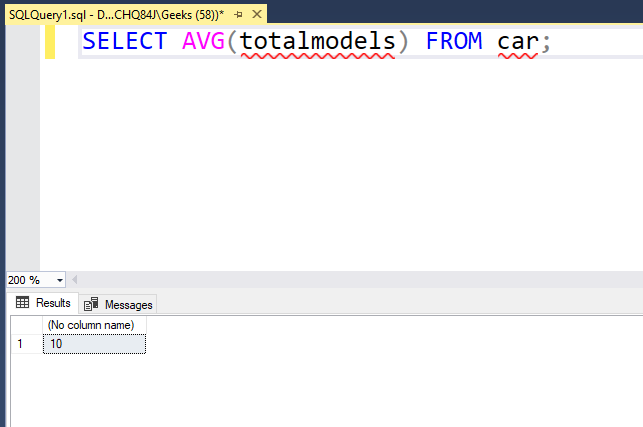# SQL Query to Find the Average Value in a Column

• Difficulty Level : Basic
• Last Updated : 24 Mar, 2022

In this article, we are going to see how to find the average value in a column in SQL.  A column in the SQL table is the vertical catalog structure. In this article, we will be using the Microsoft SQL Server as our database.

For the purpose of example, we will be creating a sample table and performing the same operations on it.

### Table Definition:

We have the following car table in our database :

```CREATE TABLE car (
companyid integer ,
companyname varchar(20) ,
totalmodels integer )```The above query creates a car table for us.

To insert values into the table we need to use the insert statement. Use the below statement to add data to the car table:

```INSERT INTO car values(1,'maruti  suzuki',10);
INSERT INTO car values(2,'tata',12);
INSERT INTO car values(3,'volkswagen',8);```The above query will add the data to the car table.

Note: We have to insert values according to the table created. For example, we created a car table with companyid as integer, companyname as varchar, and totalmodels as an integer. So, we need to insert an integer, a character, and an integer else we may get an error.

To verify the contents of the table use the below statement:

`SELECT * FROM car;`

This will show us our created table as shown below:### Average of all values in a column

For this, we need to use avg() function. We have to pass the column name as a parameter. The avg() function has the following syntax:

`SELECT AVG( column_name ) FROM table_name;`
• The avg() function can be used with the SELECT query for retrieving data from a table.

The below query can be used to find the average of the totalmodels column of our cars table:

`SELECT AVG(totalmodels) FROM car;`

Output :Let’s verify the output. The average of 10, 12, and 18 is 10.  Hence, we have successfully found out the average of all values n a column of a table.

My Personal Notes arrow_drop_up